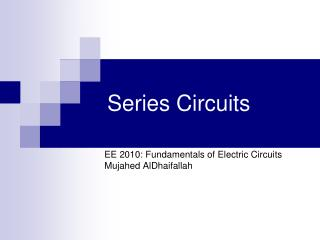Download PresentationSeries Circuits

Series Circuits - PowerPoint PPT Presentation

Series Circuits. EE 2010: Fundamentals of Electric Circuits Mujahed AlDhaifallah. Is this a series circuit?. R 1. R 2. Is this a series circuit?. R 1. R 3. R 2. Is this a series circuit?. R 1. R 3. R 2. Series Circuit. Two elements are in series ifI am the owner, or an agent authorized to act on behalf of the owner, of the copyrighted work described.
Download PresentationSeries Circuits

Download Policy: Content on the Website is provided to you AS IS for your information and personal use and may not be sold / licensed / shared on other websites without getting consent from its author.While downloading, if for some reason you are not able to download a presentation, the publisher may have deleted the file from their server.

- - - - - - - - - - - - - - - - - - - - - - - - - - E N D - - - - - - - - - - - - - - - - - - - - - - - - - -
Presentation Transcript
1. Series Circuits EE 2010: Fundamentals of Electric Circuits Mujahed AlDhaifallah

2. Is this a series circuit? R1 R3 R2

3. Is this a series circuit? R1 R3 R2

4. Series Circuit • Two elements are in series if • They have only one terminal in common. • The common point in the two elements is not connected to a third current carrying element.

5. Resistance • Resistance is proportional to length length direction of current flow

6. Resistance • R = ρL/A • ρ is the resistivity of the material (units?)

7. length length direction of current flow direction of current flow Resistance • What happens if two elements are connected back to back?

8. Resistance • R = ρ (L1+L2)/A • R = R1 + R2 • The total resistance of a series circuit is the sum of all the resistances in the path

9. R1 R2 Resistance • The resistance seen by the source • R=R1+R2 • The two circuits on the right are equivalent R1+R2

10. Voltage Drop? • The current through each resistor is calculated by the Ohm’s law • =V1/R1 • Where V1 is the voltage across the resistor. • =V/RT • Where RT is the total resistance in the circuit. • V1 = VxR1/RT

11. Power? • Power dissipated in each resistor • P1 = V12/R1 • P1 = (V2/RT2)x R1 • Total power = V2/RT = P1 + P2 + …

12. Voltage Sources in Series • Voltage sources can be connected in series to increase or decrease the total voltage applied to the system. • Net voltage is determined by summing the sources having the same polarity and subtracting the total of the sources having the opposite polarity.

13. Kirchhoff’s Voltage Law • The algebraic sum of the potential rises and drops around a closed loop is zero.

14. R1 R2 KVL • V + V1+V2 = 0 • Can anyone prove this mathematically? V1 V V2

15. Voltage Divider Rule • In a series circuit the voltage across the resistive elements will divide as the magnitude of the resistors

16. Interchanging Series Elements • Elements of a series circuit can be interchanged without affecting the total resistance, current, or power to each element. • In the Figures below, resistors 2 and 3 are interchanged without affecting the total resistance

17. This is not a loop. Or is it? Ground Terminal

18. This is not a loop. Or is it? Ground terminal means that the two points are both connected to ground and are at a zero potential. So this is a loop. Ground Terminal

19. Internal Resistances • The ideal voltage source has no internal resistance and an output voltage of V volts with no load or full load • Every practical voltage source (generator, battery, or laboratory supply) has some internal resistance. • Voltage across the internal resistance lowers the source output voltage when a load is connected. • For any chosen interval of voltage or current, the magnitude of the internal resistance is given by Rint = VL / IL

20. Parallel Circuits EE 2010: Fundamentals of Electric Circuits Mujahed AlDhaifallah

21. Are these resistors in Parallel?

22. Are these resistors in Parallel?

23. Are these resistors in Parallel?

24. Parallel Elements • Two Elements, branches or networks are in parallel if they have two points in common.

25. Resistance • Resistance is inversely proportional to the cross sectional area direction of current flow

26. direction of current flow direction of current flow Resistance • Resistance is inversely proportional to the cross sectional area

27. Resistance • R = ρL/(A1+A2) • Solving in terms of R1 and R2 gives • 1/R = 1/R1 + 1/R2 • The total value of the resistance is always smaller than the smallest resistance

28. Resistance, etc. • The total resistance will decrease with each new added parallel branch • The voltage across each resistor is the same • The total current is the sum of all the branches • The total power dissipated is the sum of power dissipated in all resistors.

29. Kirchhoff’s Current Law • KCL states that the algebraic sum of the currents entering and leaving a point or junction is zero. • i1+i2+i3+i4=0 i1 i2 i3 i4

30. Current Divider Rule • For parallel elements of different value the current will split with a ratio equal to the inverse of their resistor value